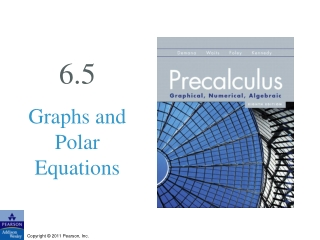DownloadDownload Presentation6.5

# 6.5

Download Presentation## 6.5

- - - - - - - - - - - - - - - - - - - - - - - - - - - E N D - - - - - - - - - - - - - - - - - - - - - - - - - - -
##### Presentation Transcript

1. 6.5 Graphs and Polar Equations

2. What you’ll learn about • Polar Curves and Parametric Curves • Symmetry • Analyzing Polar Curves • Rose Curves • Limaçon Curves • Other Polar Curves … and why Graphs that have circular or cylindrical symmetry often have simple polar equations, which is very useful in calculus.

3. Symmetry The three types of symmetry figures to be considered will have are: 1. The x-axis (polar axis) as a line of symmetry. 2. The y-axis (the line θ = π/2) as a line of symmetry. 3. The origin (the pole) as a point of symmetry.

4. Symmetry Tests for Polar Graphs The graph of a polar equation has the indicated symmetry if either replacement produces an equivalent polar equation. To Test for SymmetryReplaceBy 1. about the x-axis (r,θ) (r,–θ) or (–r, π–θ) 2. about the y-axis (r,θ) (–r,–θ) or (r, π–θ) 3. about the origin (r,θ) (–r,θ) or (r, π+θ)

5. Example Testing for Symmetry

6. Example Testing for Symmetry

7. Rose Curves

8. Limaçon Curves

9. Example Analyzing a Limaçon Curve

10. Example Analyzing a Limaçon Curve

11. Spiral of Archimedes

12. Lemniscate Curves